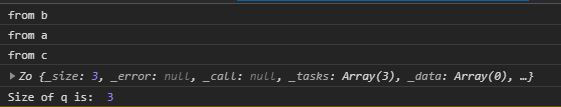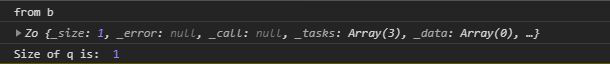# D3.js queue.defer() Function

The queue.defer() function in d3.js is used to add the asynchronous task callback to the queue. Where the task is a function to be executed. The callback must be executed when the task got finished.

Syntax:

```queue.defer(task[, arguments…]);
```

Parameters: This function accepts a single parameter as mentioned above and described below:

• task: It is a function that is to be executed to perform a particular task.

Return Value: This function returns the object.

Below given are a few examples of the above function.

Example 1: When the size of the queue is equal to the number of queue.defer() calls.

## HTML

 `  ` `<``html` `lang``=``"en"``>  ` `<``head``>  ` `    ``<``meta` `charset``=``"UTF-8"``>  ` `    ``<``meta` `name``=``"viewport"` `            ``path1tent="``width``=``device``-width,  ` `                       ``initial-scale``=``1``.0">  ` `    ``<``title``>Document  ` `  ` `<``style``> ` `  ` `<``body``>  ` `  ``<``script` `src` `=  ` `"https://d3js.org/d3.v4.min.js"``>  ` `  ``  ` `  ``<``script``> ` `    ``function a(){ ` `      ``console.log("from a") ` `    ``} ` `    ``function b(){ ` `      ``console.log("from b") ` `    ``} ` `    ``function c(){ ` `      ``console.log("from c") ` `    ``} ` `    ``let q=d3.queue(3) ` `    ``// Calling defer three times ` `    ``q.defer(b) ` `    ``q.defer(a) ` `    ``q.defer(c) ` `    ``console.log(q) ` `    ``console.log("Size of q is: ",q._size) ` `  ``  ` `  ` ` `

Output:Example 2: When the size of the queue is less than the queue.defer() calls.

## HTML

 `  ` `<``html` `lang``=``"en"``>  ` `<``head``>  ` `    ``<``meta` `charset``=``"UTF-8"``>  ` `    ``<``meta` `name``=``"viewport"` `            ``path1tent="``width``=``device``-width,  ` `                       ``initial-scale``=``1``.0">  ` `    ``<``title``>Document  ` `  ` `<``style``>  ` `  ` `<``body``>  ` `  ``<``script` `src` `=  ` `"https://d3js.org/d3.v4.min.js"``>  ` `  ``  ` `  ``<``script``> ` `    ``function a(){ ` `      ``console.log("from a") ` `    ``} ` `    ``function b(){ ` `      ``console.log("from b") ` `    ``} ` `    ``function c(){ ` `      ``console.log("from c") ` `    ``} ` `    ``let q=d3.queue(1) ` `    ``// Calling defer three times but it will add only function ` `    ``// call b because the size of the queue is one. ` `    ``q.defer(b) ` `    ``q.defer(a) ` `    ``q.defer(c) ` `    ``console.log(q) ` `    ``console.log("Size of q is: ",q._size) ` `  ``  ` `  ` ` `

Output:My Personal Notes arrow_drop_upCheck out this Author's contributed articles.

If you like GeeksforGeeks and would like to contribute, you can also write an article using contribute.geeksforgeeks.org or mail your article to contribute@geeksforgeeks.org. See your article appearing on the GeeksforGeeks main page and help other Geeks.

Please Improve this article if you find anything incorrect by clicking on the "Improve Article" button below.

Article Tags :

Be the First to upvote.

Please write to us at contribute@geeksforgeeks.org to report any issue with the above content.